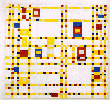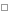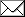Jürgen Böhms Heimatseiten - WissenschaftLispComputer-algebrasurfxpgraphicssoftwareasxpgraphicssoftwareNumericsMathematikPhysikInformatikdpack8 Package
fundamental domains calculator
sheafCohJB
compCohX
CompCoh
Resolution graphs of embedded curves

## The dpack system for lie symmetry analysis

### Introduction

#### Features

The maple package dpack is part of my work on a thesis with the title

Janetbasen und Algorithmen zur Lösung gewöhnlicher Differentialgleichungen 2. und 3. Ordnung mittels Symmetrieanalyse
Janetbases and Algorithms for Solution of ODEs of 2nd and 3rd Order by Symmetry-Analysis

which I started working on in June 1996 as member of the Group CADE at the german
GMD - National Research Center for Information Technology.

It represents a comprehensive collection of algorithms and datatypes useful for lie-symmetry analysis of ODEs and PDEs with features like

• computation of Janet-Bases
• definition of arbitrary termorderings for Janet-Base computations
• special cases of heavy-duty nonlinear differential-groebner base computation
• computation of determining systems of symmetries
• computation of prolongations
• computing invariants and differential invariants
• determining Lie-Group-Type of explicitly or implicitly given transformation groups
• calculating structure constants from the LPDE representing a lie-algebra of transformations
• solving systems of LPDE exactly by Janet-Base computations
• special fast symbolic algorithms for systems of 1st order LPDE
• algorithms for various kinds of differential elimination
• several common lie algebra calculations for implicitly given lie algebras of transformations, for example Killing-Form, Solvable-Radical
• classification of the symmetry group of an ode of order 2 or 3

#### Ease of use

When I designed dpack my goals were:

• to allow easy handling of all the formulas necessary to do the required computations
• overall good system integration: the user should not be forced to use uncommon abbbreviations, functions or representations that are incompatible to the way the expressions are represented and handled in the other routines of the surrounding maple-system
• output should look naturally and be easily understandable
• input should be concise, with as few keystrokes as necessary

#### Requirements

• Maple 8
• contains some system dependent routines tailored for unix/linux machines

It is available as a git repository at Github:

Installation instructions are in the accompanying README-File

If you are - even to the slightest extent - interested in using the software or just playing a bit with it:

Feel free to contact me about any problems encountered, or if you need help in installing the system or getting it to run on your computer (there have to be some pathnames changed in the sources probably).

There are online-help pages (made for the maple help system) included that become automatically activated, when the system is started - and I will make available some maple-worksheets showing example calculations ASAP.

# The funddomain routines

The funddomain routines are made for computing fundamental domains of congruence subgroups of SL2(Z) in the upper half plane.

The main routines are

gensToGroup ( genlis, oper )

where

• genlis is a list of maple objects
• oper is a two argument function implementing group operation on the elements of genlis

gensToGroup computes the list of group elements generated by genlis under oper

generateFundDomain ( quotGroup )

where quotGroup is a list of 2x2 matrices forming a group in SL2(Z/NZ). They represent the congruence subgroup GAMMA which is its preimage in SL2(Z).

generateFundDomain computes a list of pairs funddomain, each consisting of

• a matrix in SL2(Z) and
• a triple of indices

Together they describe a fundamental domain for the abovementioned congruence subgroup.

More precisely the fundamental domain is the union of the translates of the canonical fundamental domain FF for SL2(Z) with all the matrices in funddomain.

Additionally the indices give glueing data for the borders of the translated FF domain.

The domain FF has a T-, T^(-1)- and a S-side where an "A-Side" means the intersection of FF with A FF. The three indices tell to which triangle of the fundamental domain of GAMMA - represented as the list funddomain - the T,T^-1 and S adjacent triangle are equivalent modulo GAMMA. A negative value means, the adjacent triangle is by itself in funddomain.

generateFundDomain2 ( funddom, quotGroup )

where

• funddom is the result of applying generateFundDomain on a congruence subgroup GAMMA1 which is a subgroup of GAMMA described by quotGroup
• quotGroup is a list of 2x2 matrices forming a group in SL2(Z/NZ). They represent the congruence subgroup GAMMA which is their preimage in SL2(Z).

generateFundDomain2 computes a list funddom1 of pairs belonging to the congruence group GAMMA. The result has the same format as in generateFundDomain.

Additionally it computes a mapping of the triangles of funddom to those computed for GAMMA (that is funddom1). This mapping represents the canonical map of GAMMA1\upper-half-plane to GAMMA\upper-half-plane.

generateRhoRels ( funddom )
generateIRels ( funddom )
generateInftyRels ( funddom)

take a list as returned by generateFundDomain as argument and compute a list of points overlying rho, I and infinity in SL2(Z)\upper-half-plane, together with their multiplicities.

Returned is a list of lists of indices into funddom. Each element of that list corresponds to a point over rho, I, infinity respectively. The cardinality of the element (which is a list by itself) is the "Verzweigungsindex" (e).

The meaning of the indices in the element-list is as follows:

If one takes the indices in the list representing a point over rho (resp. I, infinity) and selects by these indices the matrices from the list funddom (e.g. point equals [i1, i2, i3], corresponding matrices are funddom[i1], funddom[i2], funddom[i3] ) then applying these matrices to the point rho (resp. I, infinity) gives the points of the fundamental domain described by funddom lying over rho (resp. I, infinity). (N.B. border identifications may apply !)

#### Requirements

• Maple 8

There it is:

The following Maple worksheet contains some example calculations:

# Computing sheaf cohomology with Singular

shjb.lib

The function sheafCohJB(m,d1,d2) computes the ranks of the cohomology groups H^i(X,m(d)) with d1 <= d <= d2 where m is a module in Singular associated to a ring R.

Then X is proj(R) and m(d) in H^i(X,m(d)) stands for the d-times twisted sheaf associated to m.

To try some examples do:

```LIB "all.lib";
LIB "sheafcoh.lib";
LIB "shjb.lib";

testJB();

def r=basering;

setring r;

sheafCoh(m1,-6,5);

sheafCohJB(m1,-6,5);

... and so on (you have the modules m0, m1, m2 predefined).
```

Comparing the results of sheafCoh and sheafCohJB, you can convince yourself (hopefully) of the correctness of my method.

Concerning the mathematical base of my algorithm it might be interesting to note that it does not use local duality but global duality in its simplest form only.

# Computing sheaf cohomology with Macaulay2

compcoh1.m2

The function compCohX(ma, deg, check) takes three arguments. The first is a module ma over a polynomial ring S=A[x_0,...,x_n] where A is an arbitrary ring. (Currently only tested with A = QQ[a]). The parameter deg gives the truncation of ma for computing the main resolution of truncate(deg,ma). The third parameter check decides if checks on degrees in the resolution complex are done. (They are done, when check is not zero).

To try some examples do:

```

(D,C,HD) = compCohX(na1, 2, 0)

(D,C,HD) = compCohX(ma1, 2, 0)

(D,C,HD) = compCohX((coker phi) ** (S1^{-1}), 2, 0)

prune HH(D ** (A/ideal(a)))

```

# A package for computing sheaf cohomology with Macaulay2

CompCoh.m2

It provides a routine to calculate the long exact cohomology sequence for a short exact sequence of coherent sheaves on P^n.

Examples

cctest.m2

# Computing algebraic deRham cohomology with Macaulay2

mon-de-rham-xt.m2

You can compute a basis of the QQ-vector space H^p_deRham(X) for the affine hypersurface X = V(H), with H in QQ[x_1..x_n] and 1 <= p <= n-1.

# Embedded resolution of curves

A routine to compute the embedded resolution of f(x,y) = 0 at the origin by iterated blowups.

```juergen@antares:~/maple/alg-geom\$ maple
|\^/|     Maple 2018 (X86 64 LINUX)
._|\|   |/|_. Copyright (c) Maplesoft, a division of Waterloo Maple Inc. 2018
<____ ____>  Waterloo Maple Inc.
|       Type ? for help.
> trvgrph(blowupentry(mkchart((y^2-x^3)^2+y^5,[x,y],1,0)));
[[E(12), E(6)]]

[[E(12), E(12)]]

[[X, E(26)]]

[[E(26), E(13)]]

[[E(26), E(12)]]

[[E(12), E(4)]]

```

E[i](j) is the exceptional curve of step i with multiplicity j, X stands for the strict transform of the curve X:f(x,y) = 0.Zuletzt geändert - 22 07 2018
Impressum